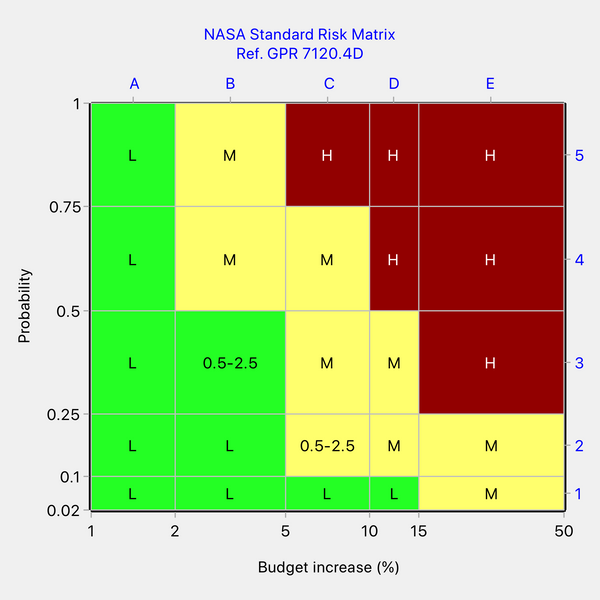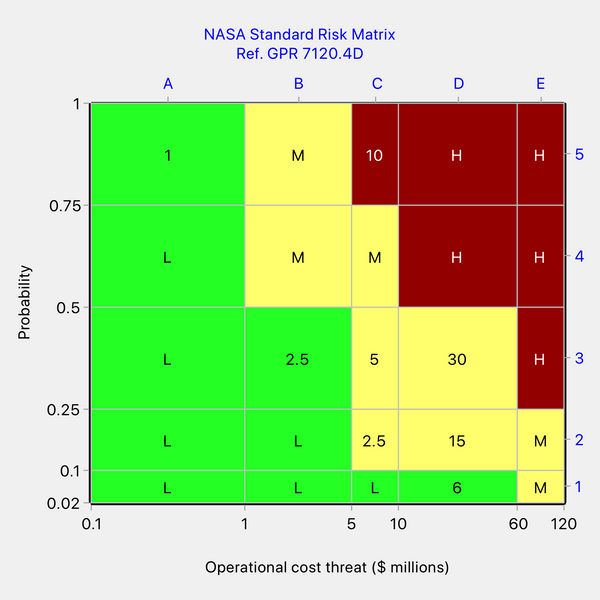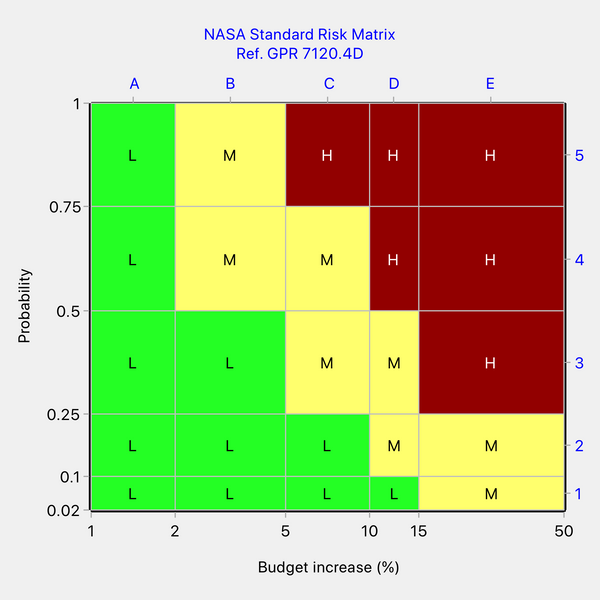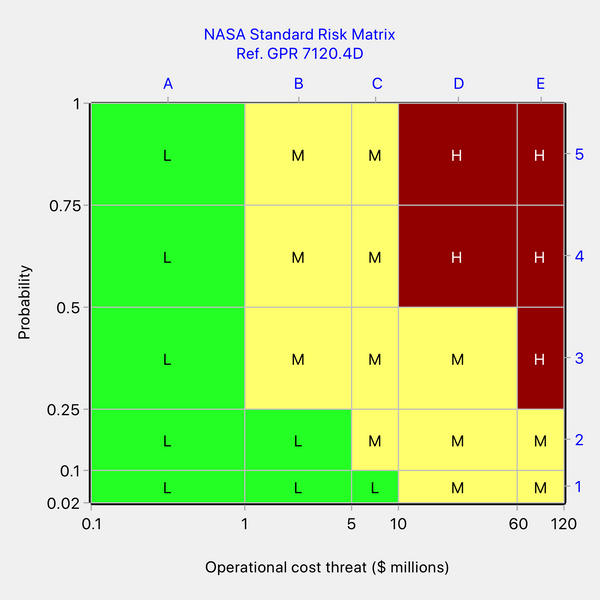# How Not to Design a Risk Matrix - NASA's Risk Matrix

NASA's activities include building spacecraft, instruments and new technology to study Earth, the Sun, the solar system and the universe.

## NASA's Standard Risk Matrix

In assessing the risks to its projects, NASA uses a standard risk matrix as shown in Figure 1 (reference NASA Goddard Procedural Requirements GPR 7120.4D, 2012).

The likelihood and consequence categories are ordinal with labels 1, 2, ... 5 along each axis. Ordinal means that the category labels do not indicate the true magnitude of the variables but only the direction of increase. We shall revise the category labels to A, B, C, ... for the horizontal axis because a reference to a cell by its column and row labels will be clearer when written as, for example, D2 rather than as 42 (D2 reminds us which digit represents the column and which represents the row). With this revision to the labels, NASA's standard matrix is as shown in Figure 1.Figure 1: NASA standard risk matrix (redrawn by us)

In case you are wondering why Figure 1 uses those particular shades of red, yellow and green to denote the risk priority levels, rather than the corresponding primary colors, it is to provide better differentiation for people with one of the three common forms of color blindness.

NASA applies the risk matrix shown in Figure 1 to different types of consequence, such as safety, technical, schedule, cost, etc. NASA associates the five consequence categories with detailed descriptions but rarely defines the consequence categories quantitatively. As we explain elsewhere in this blog, it is not possible to perform meaningful calculations with ordinal scales and, if we want to design a defensible risk matrix, we need to use ratio scales.

Fortunately for our audit of its risk matrix, NASA has provided ratio scales (as well as ordinal scales) for two types of consequence – budget increase and operational cost threat –  and we are going to use these scales to carry out logical consistency checks on their risk matrix. In making these checks, we shall assume that NASA calculates risk as the product of probability and consequence, which is by far the most common method and nothing in NASA's documentation appears to suggest anything different.

The NASA consequence categories for budget increase are <2%, 2–5%, 5–10%, 10–15%, and >15%. For operational cost threat, they are <\$1M, \$1M–\$5M, \$5M–\$10M, \$10M–\$60M, and >\$60M. Notice that the first and last categories are open at one end. We shall make some reasonable assumptions for the values of the missing endpoints since we shall want to draw the matrices to scale.

NASA likelihood categories are 2%–10%, 10%–25%, 25%–50%, 50%–75%, and >75%. We will close the last category by replacing it with 75%–100%.

## NASA's Risk Matrix Applied to Budget Increase

Figure 2 shows NASA's risk matrix when applied to budget increase and when drawn to scale. Note that we have used a logarithmic scale for the horizontal axis and a linear scale for the vertical axis. The choice of linear or logarithmic scale is made according to which is better at preventing overlapping of labels and has no effect on risk matrix design.Figure 2: NASA risk matrix for budget increase when drawn to scale

We detected an inconsistency in the risk matrix for budget increase. Look closely at cells B3 and D2. As shown in Figure 3 below, the quantitative risk in both these cells ranges from 0.5 at the bottom left corner of the cell to 2.5 at the top right corner. Yet B3 has a lower risk priority level than D2. Both cells should be of the same color since they contain an identical range of risks.Figure 3: Showing an inconsistency in NASA's risk matrix for budget increase

We conclude from Figure 3 that the coloring of the matrix is inconsistent with its underlying ratio scales in the case of the consequence type "budget increase".

## NASA's Risk Matrix Applied to Operational Cost Threat

Now let's look at the NASA standard risk matrix when applied to operational cost threat. We can find inconsistencies in this risk matrix too, but it is not as straightforward to do so as in the previous case. The matrix can be assumed to be underlain by two undisclosed iso-risk contours that define the boundaries between risk priority levels. To identify inconsistencies, we need to know NASA's strategy for determining the color of a cell split by a contour. A split cell contains some risk points that lie in the risk priority level below the contour and other points that lie in the risk priority level above the contour. We shall assume that NASA's strategy is to apply the color above the contour to the split cell. Quick Risk Matrix provides a choice of algorithms for coloring split cells, one of which is called the "round-up" algorithm. In effect, we are assuming that NASA is using the round-up algorithm. With this algorithm, the color of a split cell is determined only by the risk value at the top right corner of the cell, which is the maximum possible value for a risk point in that cell. With this assumption, we can now proceed to look for inconsistencies considering only the maximum risk value in each cell.

Figure 4 below shows NASA's matrix for operational cost threat with the maximum risk value written into several of the cells.Figure 4: NASA's risk matrix for operation cost threat with the maximum risk value written into several cells

Examining Figure 4, cell C2 (Medium risk) has a maximum value of 2.5, which is identical to the maximum value for cell B3 (Low risk) and less than the maximum value for cell D1 (Low risk). We can see that NASA is not weighting for higher consequences because D1 (Low risk) is higher consequence than C2 (Medium risk). We could resolve this inconsistency in various ways. One way would be to assume that C2 is correctly colored. Then the color of cells B3 and D1 should be the same as the color of cell C2, ie. cells B3 and D1 should be uprated from Low risk to Medium risk.

Still looking at Figure 4, cell C5 (High risk) has a maximum value of 10, which is less than the maxima for cells D3 and D2 (maxima 30 and 15, respectively, and Medium risks). Once again, we can see that NASA is not weighting for high consequence since C5 (High risk) has lower consequence than D2 or D2 (Medium risk). Again, the inconsistency could be resolved in various ways. If we assume cells D3 and D2 (Medium risk) are correctly colored, then cell C5 would need to be downrated from High to Medium risk.

## The Corrected Risk Matrices

It is emphasized that there many ways to revise the two risk matrices to make them internally consistent. We gave examples of possible corrections in the previous sections. Applying those corrections, the revised risk matrices are as shown in Figures 5 and 6 below.Figure 5: One possible revision to NASA's risk matrix for budget increase to make it self-consistentFigure 6: One possible revision to NASA's risk matrix for operational cost threat to make it self-consistent

A matrix identical to that in Figure 5 may be obtained in Quick Risk Matrix by using the round-up algorithm and iso-risk contour values of 1.25% and 5%.

A matrix identical to that in Figure 6 may be obtained in Quick Risk Matrix by using the round-up algorithm and iso-risk contour values of \$1.25M and \$30M.

Although we made both matrices self-consistent, we had to adopt different coloring patterns to do so. This illustrates that changing the axis scales of a risk matrix will, in general, also change the required coloring pattern.

## Discussion and Conclusions

We can learn many lessons from this review:

• For the two consequence types for which we were able to audit NASA's risk matrix because ratio scales had been defined, we demonstrated that the risk matrix coloring is inconsistent with the underlying ratio scales (based on the usual definition of quantitative risk as the product of probability and consequence). The anomalies cannot be explained by assuming that NASA is weighting for higher consequences.
• There may be additional design imperfections in the two matrices but it is difficult to say with certainty without knowing the design intent, in particular, the intended values of the two risk thresholds separating the three risk levels.
• When only ordinal scales are given, the associated matrix will be largely unverifiable and therefore cannot be regarded as technically defensible. This applies to most of the scales employed by NASA. Sometimes ordinal scales look like numbers (e.g. 1...5), but they are really just labels and calculations should never be performed with them.
• The same risk matrix coloring cannot be blindly assumed to apply when the underlying scales are changed. If the scales (or the risk thresholds) are changed, the appropriate coloring pattern will change in general.
• If it is desired to use the same coloring pattern with different scales and risk thresholds, this will only be possible when the axis scales and risk thresholds are changed proportionately. Arbitrary changes to the scales or thresholds will invalidate the coloring.
• Even organizations with impressive technology such as NASA are not immune from making errors when designing risk matrices.
• Errors of design can be avoided by using Quick Risk Matrix, which guarantees a self-consistent risk matrix every time.